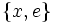# Derived subgroup of M16

This article is about a particular subgroup in a group, up to equivalence of subgroups (i.e., an isomorphism of groups that induces the corresponding isomorphism of subgroups). The subgroup is (up to isomorphism) cyclic group:Z2 and the group is (up to isomorphism) M16 (see subgroup structure of M16).
The subgroup is a normal subgroup and the quotient group is isomorphic to direct product of Z4 and Z2.
VIEW: Group-subgroup pairs with the same subgroup part | Group-subgroup pairs with the same group part| Group-subgroup pairs with the same quotient part | All pages on particular subgroups in groups

## Definition

We consider the group:$G = M_{16} = \langle a,x \mid a^8 = x^2 = e, xax = a^5 \rangle$

with$e$ denoting the identity element.

This is a group of order 16, with elements:$\{ e, a, a^2, a^3, a^4, a^5, a^6, a^7, x, ax, a^2x, a^3x, a^4x, a^5x, a^6x, a^7x \}$

We are interested in the subgroup:$H = \{ e, a^4 \}$

This is a cyclic subgroup of order two, i.e., it is isomorphic to cyclic group:Z2. The quotient group is isomorphic to the abelian group direct product of Z4 and Z2.

## Cosets

The subgroup is a normal subgroup and hence its left cosets coincide with its right cosets. The eight cosets are given below:$\{ e, a^4 \}, \{ a, a^5 \}, \{ a^2, a^6 \}, \{ a^3, a^7 \}, \{x, a^4x \}, \{ ax, a^5x \}, \{ a^2x, a^6x \}, \{ a^3x, a^7x \}$

## Complements

The subgroup has no permutable complements. Since it is a normal subgroup, this also means it has no lattice complements.

### Properties related to complementation

Property Meaning Satisfied? Explanation Comment
complemented normal subgroup normal subgroup with permutable complement No see above
permutably complemented subgroup subgroup with permutable complement No
lattice-complemented subgroup subgroup with lattice complement No
retract has a normal complement No
direct factor normal subgroup with normal complement No

## Arithmetic functions

Function Value Explanation
order of whole group 16
order of subgroup 2
index 8
size of conjugacy class 1
number of conjugacy classes in automorphism class 1

## Effect of subgroup operators

Function Value as subgroup (descriptive) Value as subgroup (link) Value as group
normalizer whole group$\langle a,x \rangle$ M16
centralizer whole group$\langle a,x \rangle$ M16
normal core the subgroup itself current page cyclic group:Z2
normal closure the subgroup itself current page cyclic group:Z2
characteristic core the subgroup itself current page cyclic group:Z2
characteristic closure the subgroup itself current page cyclic group:Z2
commutator with whole group trivial subgroup -- trivial group

## Subgroup-defining functions

The subgroup is a characteristic subgroup of the whole group and arises as a result of many subgroup-defining functions on the whole group. Some of these are given below.

Subgroup-defining function Meaning in general Why it takes this value
derived subgroup subgroup generated by all commutators between elements of the group The only commutators are the identity element and$a^4$.
socle subgroup generated by the minimal normal subgroups. For a group of prime power order$G$, this is the same as$\Omega_1(Z(G))$ -- the identity element and all elements of prime order in the center It is the unique minimal normal subgroup. The center is in fact$\langle a^2 \rangle$, and this is precisely the set of elements of order at most 2 in the center.
second agemo subgroup subgroup generated by all$(p^2)^{th}$ powers where$p$ is the underlying prime (here$p = 2$) The fourth powers are precisely the identity and$a^4$.

## Subgroup properties

### Invariance under automorphisms and endomorphisms

Property Meaning Satisfied? Explanation
normal subgroup invariant under inner automorphisms Yes Follows from its being the derived subgroup.
characteristic subgroup invariant under all automorphisms Yes Follows from its being the derived subgroup.
fully invariant subgroup invariant under all endomorphisms Yes Follows from its being the derived subgroup.
verbal subgroup generated by set of words Yes Follows from its being the derived subgroup.
image-closed characteristic subgroup image under any surjective homomorphism from whole group is characteristic in target group Yes Follows from its being a verbal subgroup.
image-closed fully invariant subgroup image under any surjective homomorphism from whole group is fully invariant in target group Yes Follows from its being a verbal subgroup.
isomorph-free subgroup no other isomorphic subgroup No$\{ x, e \}$ is another isomorphic subgroup.
isomorph-normal subgroup every isomorphic subgroup is normal No$\{ x, e \}$ is a non-normal isomorphic subgroup.
normal-isomorph-free subgroup no other isomorphic normal subgroup Yes Follows from its being the socle.
homomorph-containing subgroup contains all homomorphic images No Follows from not being isomorph-free.
1-endomorphism-invariant subgroup invariant under all 1-endomorphisms of the group Yes Follows from its being precisely the set of fourth powers.
1-automorphism-invariant subgroup invariant under all 1-automorphisms of the group Yes Follows from being 1-endomorphism-invariant.
quasiautomorphism-invariant subgroup invariant under all quasiautomorphisms Yes Follows from being 1-automorphism-invariant

## GAP implementation

The group and subgroup can be constructed using GAP's SmallGroup and DerivedSubgroup functions as follows:

G := SmallGroup(16,6); H := DerivedSubgroup(G);

The GAP display looks as follows:

gap> G := SmallGroup(16,6); H := DerivedSubgroup(G);
<pc group of size 16 with 4 generators>
Group([ f4 ])